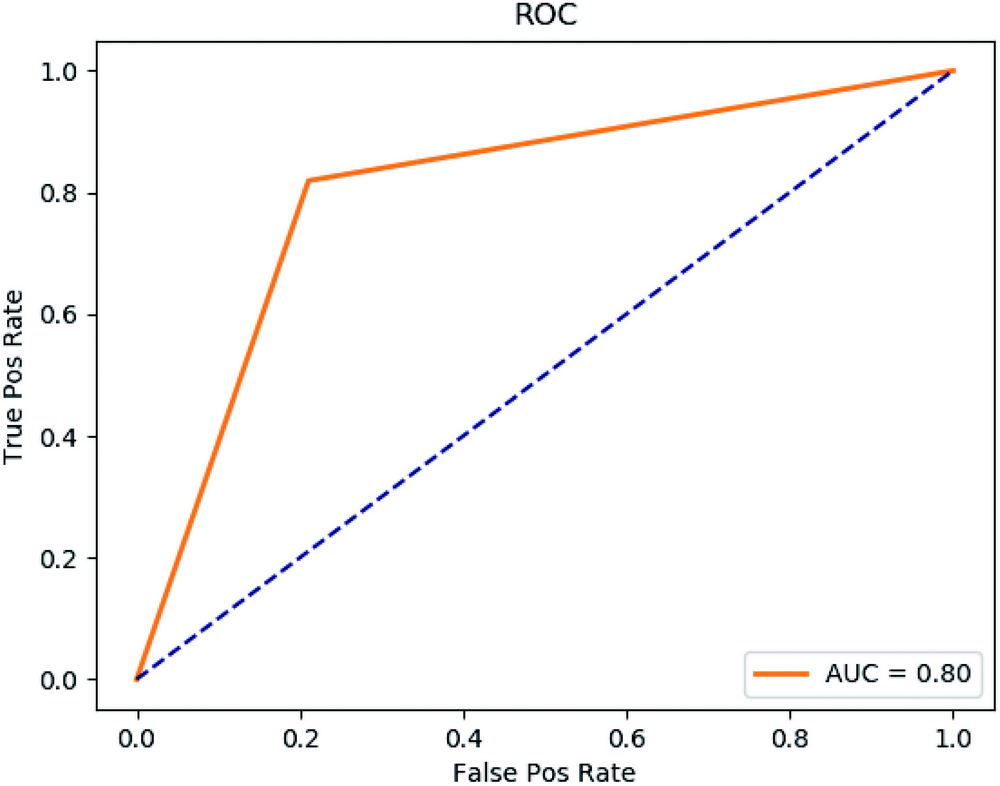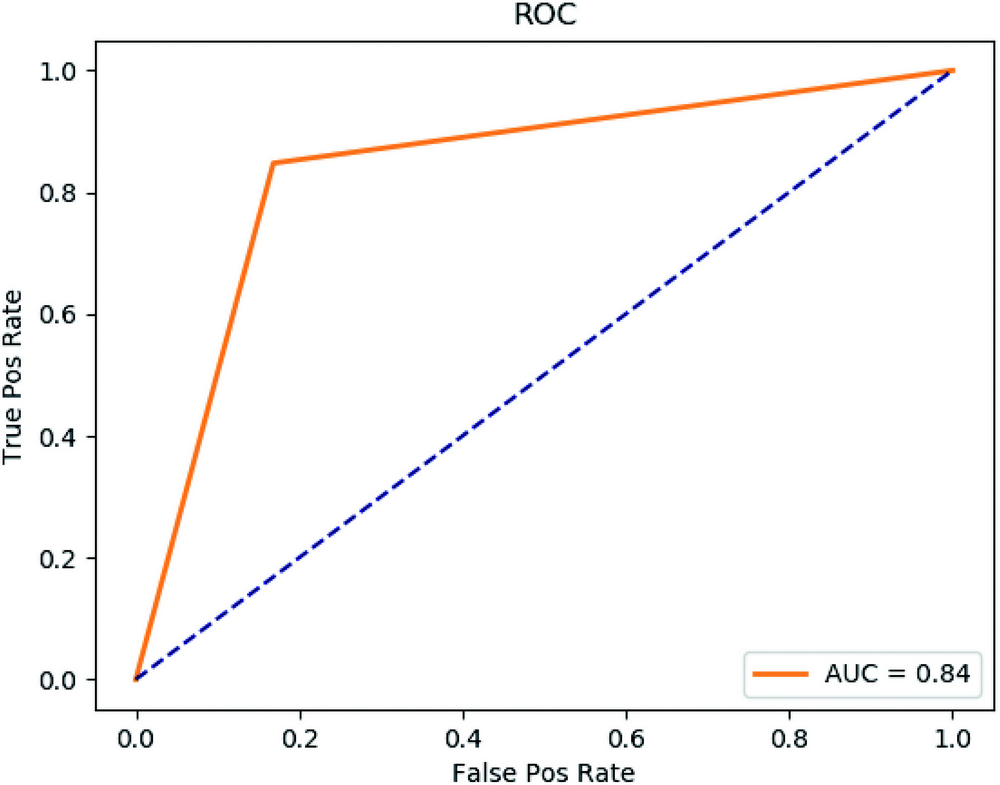# AI 安全之对抗样本入门 (20)：深度学习基础知识 1.5.1(Boosting 算法)

Boosting 系列算法的原理是在训练集用初始权重训练出一个分类器，根据分类器的表现来更新训练样本的权重，使得这些错误率高的样本在后面的训练中得到更多的重视。如此重复进行，直到分类器的数量达到事先指定的数目，最终将全部分类器通过集合策略进行整合，得到新的分类器。

x, y = datasets.make_classification(n_samples=1000,n_features=100,n_redundant=0, random_state = 1)train_X, test_X, train_Y, test_Y = train_test_split(x,                                                    y,                                                    test_size=0.2,                                                    random_state=66)clf = AdaBoostClassifier(n_estimators=100)clf.fit(train_X, train_Y)pred_Y = clf.predict(test_X)

accuracy_score:0.805f1_score:0.815165876777recall_score:0.819047619048precision_score:0.811320754717confusion_matrix:[[75 20] [19 86]]AUC:0.804260651629x, y = datasets.make_classification(n_samples=1000,n_features=100,n_redundant=0, random_state = 1)train_X, test_X, train_Y, test_Y = train_test_split(x,                                                    y,                                                    test_size=0.2,                                                    random_state=66)clf = GradientBoostingClassifier(n_estimators=100)clf.fit(train_X, train_Y)pred_Y = clf.predict(test_X)report(test_Y, pred_Y)

accuracy_score:0.84f1_score:0.847619047619recall_score:0.847619047619precision_score:0.847619047619confusion_matrix:[[79 16] [16 89]]AUC:0.839598997494